# How to Highlight Odd Number and Even Number by Conditional Format in Excel?

Suppose we have a table of numbers, and we want to highlight odd number and even number by different format settings to distinguish them, in this situation we need to know how to identify odd number and even number. Besides, we need to know how to highlight them by different formats. Actually, excel has a lot of strong functions, user can highlight odd and even number with different formats by Conditional Format function properly. This article will show you the method to identify odd and even number, and the method to highlight them.

## Part 1. How to Identify Odd and Even Number in Excel

See below table, odd numbers and even numbers exist. We can use ISODD and ISEVEN functions to identify them. See details below.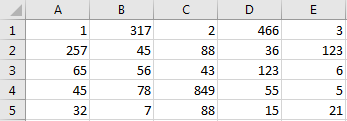Step 1: Insert a new column next to column A. Then in B1, enter the formula =ISODD(A1).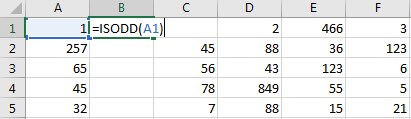Step 2: Click Enter. Verify that True is displayed in B1 properly. That means A1 value is an odd number.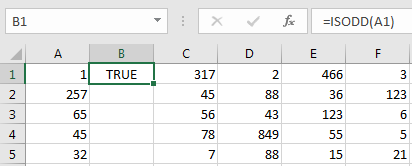Step 3: Drag the fill handler to fill cells B1:B5.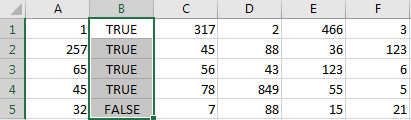Step 4: Select column B, right click and select Copy, then select column D, right click and select Insert Copied Cells.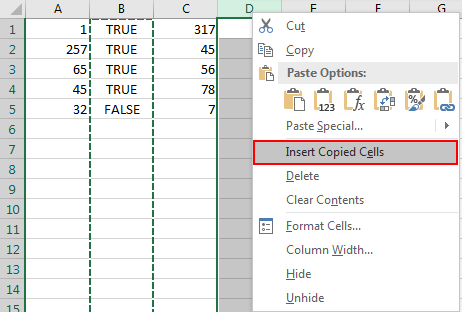Step 5: Then column B with formula will be pasted in D column, True or False value is displayed based on value in column C.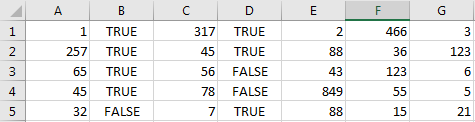Step 6: Repeat above step on other columns. Then you can do filter on column B, D etc. to filter odd number or even number and mark them with different colors.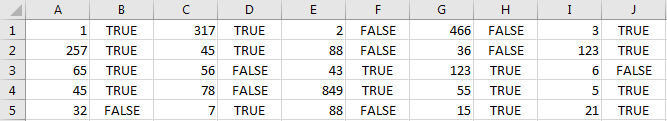Comment:

1. You can also use ISEVEN function in above steps. This time if value is even number, formula will return True.

## Part 2. How to Highlight Odd and Even Number by Conditional Format in Excel

We can identify odd and even number by above method, though we can do filter on each column and then mark them with different colors, the operation is complex and troublesome. So we need a quick and convenient way to highlight them directly.

We still use previous table to do demonstration.Step 1: First, select the range you want to do filter. In this case select A1:E5.

Step 2: In ribbon, click Home->Conditional Formatting under Styles.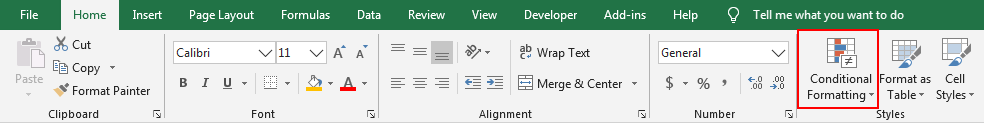Step 3: Click the arrow button on Conditional Formatting icon to load all sub menus, select New Rule in it.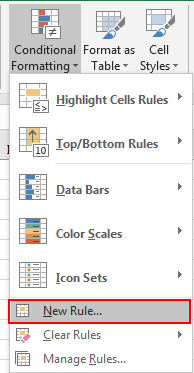Step 4: In the pops up New Formatting Rule window, in Select a Rule Type panel, select Use a formula to determine which cells to format.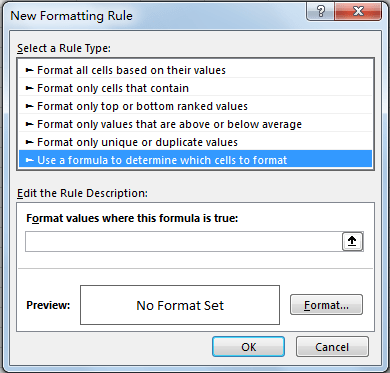Step 5: In Edit the Rule Description panel, in Format values where this formula is true textbox, enter the formula =ISODD(A1).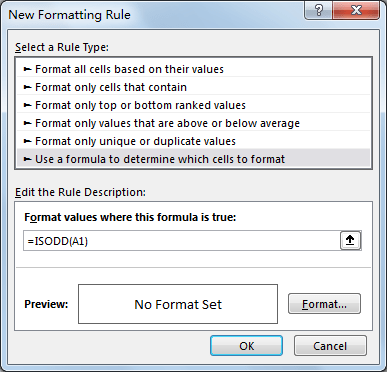Step 6: After entering the formula, click Format button to specify your cell format for highlighted cells. You can design the format by your demands. In this case, we just mark them with light blue background in cells. In Format Cells, click Fill, select Background Color, then click OK.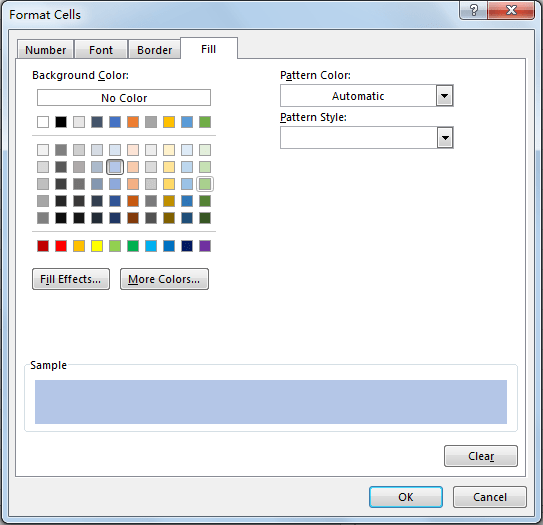Step 7: Returns to New Formatting Rule window, see the Preview. Verify that cell is filled with light blue background. Then click OK.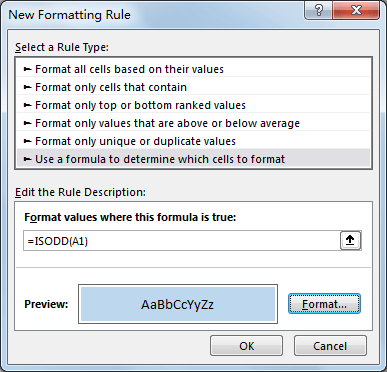Step 8: Verify that all odd numbers are marked with light blue.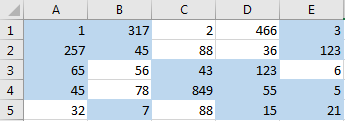Step 9: Repeat above steps, set Format values where this formula is true =ISEVEN(A1), then you can also highlight even numbers with other color.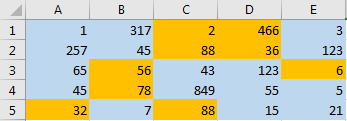Related Posts

Cap percentage values between 0 and 100

This article will talk about how to cap the percentage values between 0% and 100% in Microsoft Excel Spreadsheet or Google Sheets. If you are a newbie on Excel or google Sheets, you may be able to do this by ...

Calculate Cap Percentages to Specific Value

This article will talk about how to limit the cap percentage of a given amount to a given value in Microsoft Excel Spreadsheet or Google Sheets. If you are a newbie on Excel or google Sheets, you may be able ...

Calculate Win Loss Tie

Suppose you got a task to calculate the win, loss, and tie totals; what would you do? If you are new to Ms Excel and don't have enough experience with it, then you might do this task manually but let ...

Calculate Years Between Dates In Ms Excel

If you are an avid Ms Excel user, then you might have come across a task in which you needed to calculate the years between the dates; you might take it easy and do this task manually, which is also ...

Calculate Number of Hours between Two Times

Calculating the difference between two times might be a valuable statistic for subsequent computations or averages, whether you're producing a time sheet for staff or recording personal exercises. While Excel has a plethora of complex functions, including date and time ...

Calculate Loan Interest in Given Year

When you borrow money, you are supposed to repay it gradually. Lenders, on the other hand, want to be compensated for their services and the risk they incur by lending you money. That is, you will not just repay the ...

Calculate Interest Rate for Loan

The interest rate is the fee charged by a lender to a borrower and is expressed as a percentage of the principal—the lent amount. The interest rate on a loan is often expressed as an annual percentage rate, abbreviated as ...

Calculate Interest for Given Period

Using the IPMT function in Excel, we can compute the interest payment on any loan. This step-by-step tutorial will guide Excel users of all skill levels through the process to calculate interest for given period. Finally, the formula: =IPMT(B3/12,1,B5,-B2) The ...

How To Use Excel GCD Function

This post will guide you how to use Excel GCD function with syntax and examples in Microsoft excel. Description The Excel GCD function Returns the greatest common divisor of two or more integers. So you can use the GCD function ...

Calculate A Ratio From Two Numbers In Excel

In elementary mathematics, a ratio is a connection or comparison between two or more integers. For example, ratios are often expressed as ":" to demonstrate the relationship between two numbers. You would think that manually calculating a ratio from two ...

Sidebar